### Day 27 - Difference of Squares - 02.17.15

• Unit 3 Test this Friday, February 20th!
• Go over Quiz 3-1

Bell Ringer

1. Simplify the following: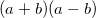1.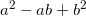2.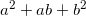3.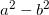4.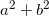5. none of the above

2. Factor the following:

1.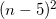2.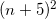3.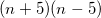4. all of the above

5. none of the above

3. Factor the following:1.2.3.4. all of the above

5. none of the above

4. Factor the following: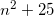1.2.3.4. all of the above

5. none of the above

Review
• Prerequisites
• Prime Numbers
• Composite Numbers
• Factor
• Distributive Property
• Factoring (video 1) (video 2)
• Classifying Numbers as Prime or Composite
• Prime Factorization of a Positive Integer
• Prime Factorization of a Negative Integer
• Prime Factorization of a Monomial
• Finding the GCF of an Integer
• Finding the GCF of a Monomial
• Apply Factoring to Solve Problems
• Factoring and Distributive Property (video)
• Zero Product Property (video)
• Factor polynomials using the Distributive Property
• Factor by grouping
• Solve quadratic equations of the following form:
• Factoring Trinomials
• Factor trinomials of the form
• Solve equations of the form
• Factor trinomials of the form
• Solve equations of the form

Lesson

Exit Ticket
• Posted on board at the end of the block
Lesson Objective(s)
• How can factoring the difference of squares be used to solve equations?
Skills
• Factor using difference of squares
• Solve equations using graphing

#### In-Class Help Requests

Standard(s)
• CC.9-12.A.SSE.1a Interpret parts of an expression, such as terms, factors, and coefficients.

Mathematical Practice(s)
• #1 - Make sense of problems and persevere in solving them
• #2 - Reason abstractly and quantitatively
• #7 - Look for and make use of structure

Past Checkpoints

Unit Skills
1. Classifying Numbers as Prime or Composite
2. Prime Factorization of a Positive Integer
3. Prime Factorization of a Negative Integer
4. Prime Factorization of a Monomial
5. Finding the GCF of an Integer
6. Finding the GCF of a Monomial
7. Factor Applications
8. Factor polynomials using the Distributive Property
9. Factor by grouping
10. Solve quadratic equations of the following form:
11. Solve factored equations using Zero Product Property
12. Factor trinomials of the form
13. Solve equations of the form
14. Factor trinomials of the form
15. Solve equations of the form
16. Factor using difference of squares
17. Solve equations using graphing